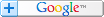# Sum Excel Ranges Diagonally

## Sum: Left-to-right | Right-to-left | Top-down | Bottom-up

Got any Excel Questions? Excel Help

NEW & FREE! Excel Custom Functions Add-in (has this and many other functions within) | Excel List to Table Creator Create an classic Excel table from data in a single column list. | Fill Blanks Excel Add-in Fill blanks in a list with the cell above

## Diagonal Sum

Ever wanted to sum some cells in Excel, but with a twist. Sum cells diagonally top-to-bottom and vice versa, left-to-right and vice versa. This code will give Excel that ability. As with nearly all Custom Functions for Excel, it pays to keep the ranges used as small as possible.

```Function SumDiagonal(rRange As Range, LrRl As String, Optional BsrtBottom As Boolean) As Single

'''''''''''''''''''''''''''''''''''''''''''''''''''''''''''''''''''''''''''
'WRITTEN BY OZGRID.COM

'Sum a range cells diagonally. Summation occurs left-to-right or right-to-left _
top-to-bottom (default) or bottom-to-top

'rRange should be as small as possible for efficieny
Dim lRows As Long, lCol As Long, lLoopR As Long, lloopC As Long
Dim sTot As Single

lRows = rRange.Rows.Count
lCol = rRange.Columns.Count
lloopC = 1
With WorksheetFunction
'Left to right, top to bottom
If UCase(LrRl) = "LR" And BsrtBottom = False Then
For lLoopR = 1 To lRows
sTot = .Sum(rRange(lLoopR, lloopC), sTot)
lloopC = lloopC + 1
Next lLoopR
'Right to left, top to bottom
ElseIf UCase(LrRl) = "RL" And BsrtBottom = False Then
lloopC = lCol
For lLoopR = 1 To lRows
sTot = .Sum(rRange(lLoopR, lloopC), sTot)
lloopC = lloopC - 1
If lloopC = 0 Then GoTo Result
Next lLoopR
'Right to left, bottom top
ElseIf UCase(LrRl) = "RL" And BsrtBottom = True Then
lloopC = lCol
For lLoopR = lRows To 1 Step -1
sTot = .Sum(rRange(lLoopR, lloopC), sTot)
lloopC = lloopC - 1
If lloopC = 0 Then GoTo Result
Next lLoopR
'Left to right, bottom top
ElseIf UCase(LrRl) = "LR" And BsrtBottom = True Then
lloopC = lCol
For lLoopR = lRows To 1 Step -1
sTot = .Sum(rRange(lLoopR, lloopC), sTot)
lloopC = lloopC - 1
If lloopC = 0 Then GoTo Result
Next lLoopR
End If
End With
Result:
SumDiagonal = sTot
End Function
```

### Example usage;

=SumDiagonal(A2:G100,lr) left-to-right/top-to-bottom OR =SumDiagonal(A2:G100,rl,TRUE) right-to-left/bottom-to-top

Excel Dashboard Reports & Excel Dashboard Charts 50% Off

Become an ExcelUser Affiliate & Earn Money

Special! Free Choice of Complete Excel Training Course OR Excel Add-ins Collection on all purchases totaling over \$64.00. ALL purchases totaling over \$150.00 gets you BOTH! Purchases MUST be made via this site. Send payment proof to [email protected] 31 days after purchase date.

# Excel VBA Video Training/ EXCEL DASHBOARD REPORTSSearch Tips FREE Excel Help